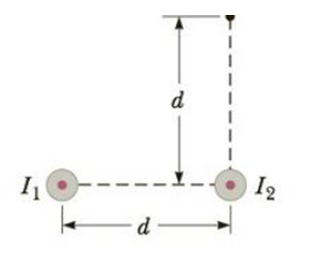Chapter 19, Problem 51P

Chapter
Section
Textbook Problem

Two long, parallel wires carry currents of I1 = 3.00 A and I2 = 5.00 A in the direction indicated in Figure P19.50. (a) Find the magnitude and direction of the magnetic field at a point midway between the wires (d = 20.0 cm). (b) Find the magnitude and direction of the magnetic field at point P, located d = 20.0 cm above the wire carrying the 5.00-A current.Figure P19.50

To determine
The magnetic field at point P .

Explanation

Given info:

The wire along x-axis is carrying a current of 7.00A . The wire along y-axis is carrying a current of 6.00A . The point P is located at x=4.00m and.

Explanation:

The magnetic field of a long straight wire is given by,

B=μ0I2πr

• μ0 is the permeability of free space
• I is the current
• r is the distance of the point from the straight conductor

Consider the positive direction of the magnetic field to be out of the page.

The magnetic field at P due to the wire along x-axis will be,

Bx=μ0Ix2πrx

• Ix is the current in the wire along the x-axis
• rx is the distance of the point P from the x-axis

Bx is positive since it is pointing out of the page.

The magnetic field at P due to the wire along y-axis will be,

By=μ0Iy2πry

• Iy is the current in the wire along the y-axis
• ry is the distance of the point P from the y-axis

By is negative since it is pointing into the page

Still sussing out bartleby?

Check out a sample textbook solution.

See a sample solution

The Solution to Your Study Problems

Bartleby provides explanations to thousands of textbook problems written by our experts, many with advanced degrees!

Get Started